6.2

### 7Plot Utilities

 (require plot/utils) package: plot-lib

#### 7.1Formatting

procedure

 (digits-for-range x-min x-max [ base extra-digits]) → exact-integer?
x-min : real?
x-max : real?
base : (and/c exact-integer? (>=/c 2)) = 10
extra-digits : exact-integer? = 3
Given a range, returns the number of decimal places necessary to distinguish numbers in the range. This may return negative numbers for large ranges.

Examples:

 > (digits-for-range 0.01 0.02) 5 > (digits-for-range 0 100000) -2

 procedure(real->plot-label x digits [scientific?]) → string? x : real? digits : exact-integer? scientific? : boolean? = #t
Converts a real number to a plot label. Used to format axis tick labels, point-labels, and numbers in legend entries.

Examples:

 > (let ([d  (digits-for-range 0.01 0.03)]) (real->plot-label 0.02555555 d))

".02556"

> (real->plot-label 2352343 -2)

"2352300"

> (real->plot-label 1000000000.0 4)

"1×10⁹"

> (real->plot-label 1000000000.1234 4)

"(1×10⁹)+.1234"

 procedure(ivl->plot-label i [extra-digits]) → string? i : ivl? extra-digits : exact-integer? = 3
Converts an interval to a plot label.

If i = (ivl x-min x-max), the number of digits used is (digits-for-range x-min x-max 10 extra-digits) when both endpoints are rational?. Otherwise, it is unspecified—but will probably remain 15.

Examples:

 > (ivl->plot-label (ivl -10.52312 10.99232)) "[-10.52,10.99]" > (ivl->plot-label (ivl -inf.0 pi)) "[-inf.0,3.141592653589793]"

 procedure(->plot-label a [digits]) → string? a : any/c digits : exact-integer? = 7
Converts a Racket value to a label. Used by discrete-histogram and discrete-histogram3d.

 procedure x : real? e : exact-integer?
Like real->decimal-string, but removes any trailing zeros and any trailing decimal point.

procedure

 (real->decimal-string* x min-digits [ max-digits]) → string?
x : real?
min-digits : exact-nonnegative-integer?
max-digits : exact-nonnegative-integer? = min-digits
Like real->decimal-string, but accepts both a maximum and minimum number of digits.

Examples:

 > (real->decimal-string* 1 5 10) "1.00000" > (real->decimal-string* 1.123456 5 10) "1.123456" > (real->decimal-string* 1.123456789123456 5 10) "1.1234567891"
Applying (real->decimal-string* x min-digits) yields the same value as (real->decimal-string x min-digits).

 procedure x : exact-integer?
Converts an integer into a string of superscript Unicode characters.

Example:

 > (integer->superscript -1234567890) "⁻¹²³⁴⁵⁶⁷⁸⁹⁰"
Systems running some out-of-date versions of Windows XP have difficulty with Unicode superscripts for 4 and up. Because integer->superscript is used by every number formatting function to format exponents, if you have such a system, Plot will apparently not format all numbers with exponents correctly (until you update it).

}

#### 7.2Sampling

procedure

 (linear-seq start end num [ #:start? start? #:end? end?]) → (listof real?)
start : real?
end : real?
num : exact-nonnegative-integer?
start? : boolean? = #t
end? : boolean? = #t
Returns a list of uniformly spaced real numbers between start and end. If start? is #t, the list includes start. If end? is #t, the list includes end.

This function is used internally to generate sample points.

Examples:

> (linear-seq 0 1 5)

'(0 1/4 1/2 3/4 1)

> (linear-seq 0 1 5 #:start? #f)

'(1/9 1/3 5/9 7/9 1)

> (linear-seq 0 1 5 #:end? #f)

'(0 2/9 4/9 2/3 8/9)

> (linear-seq 0 1 5 #:start? #f #:end? #f)

'(1/10 3/10 1/2 7/10 9/10)

> (define xs (linear-seq -1 1 11))
> (plot (lines (map vector xs (map sqr xs))))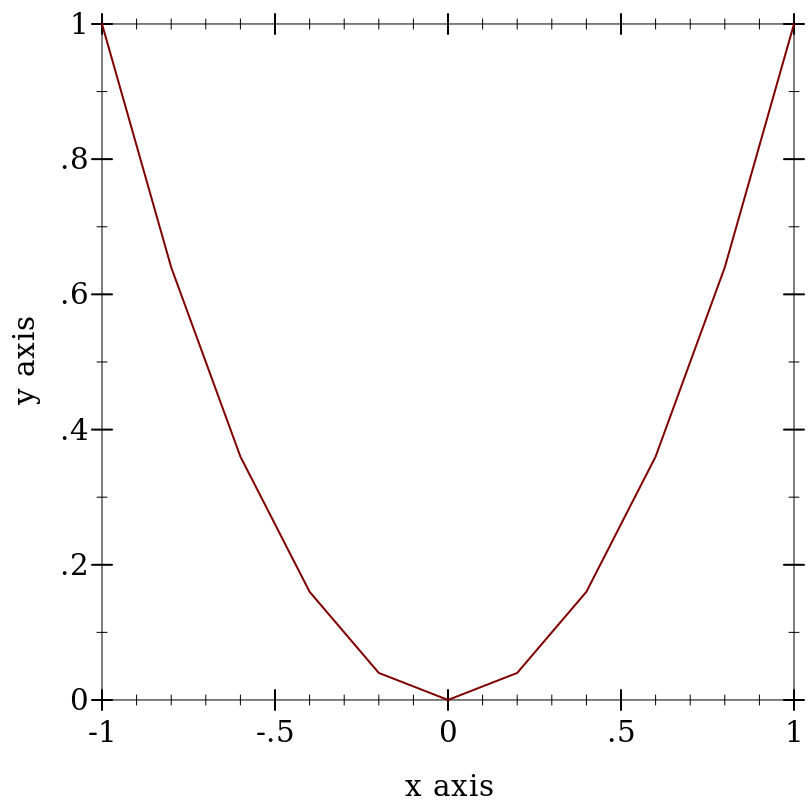procedure

 (linear-seq* points num [ #:start? start? #:end? end?]) → (listof real?)
points : (listof real?)
num : exact-nonnegative-integer?
start? : boolean? = #t
end? : boolean? = #t
Like linear-seq, but accepts a list of reals instead of a start and end. The #:start? and #:end? keyword arguments work as in linear-seq. This function does not guarantee that each inner value will be in the returned list.

Examples:

 > (linear-seq* '(0 1 2) 5) '(0 1/2 1 3/2 2) > (linear-seq* '(0 1 2) 6) '(0 2/5 4/5 6/5 8/5 2) > (linear-seq* '(0 1 0) 5) '(0 1/2 1 1/2 0)

procedure

 (nonlinear-seq start end num transform [ #:start? start? #:end? end?]) → (listof real?)
start : real?
end : real?
num : exact-nonnegative-integer?
transform : axis-transform/c
start? : boolean? = #t
end? : boolean? = #t
Generates a list of reals that, if transformed using transform, would be uniformly spaced. This is used to generate samples for transformed axes.

Examples:

> (linear-seq 1 10 4)

'(1 4 7 10)

> (nonlinear-seq 1 10 4 log-transform)

'(1.0 2.154434690031884 4.641588833612779 10.000000000000002)

 > (parameterize ([plot-x-transform  log-transform]) (plot (area-histogram sqr (nonlinear-seq 1 10 4 log-transform))))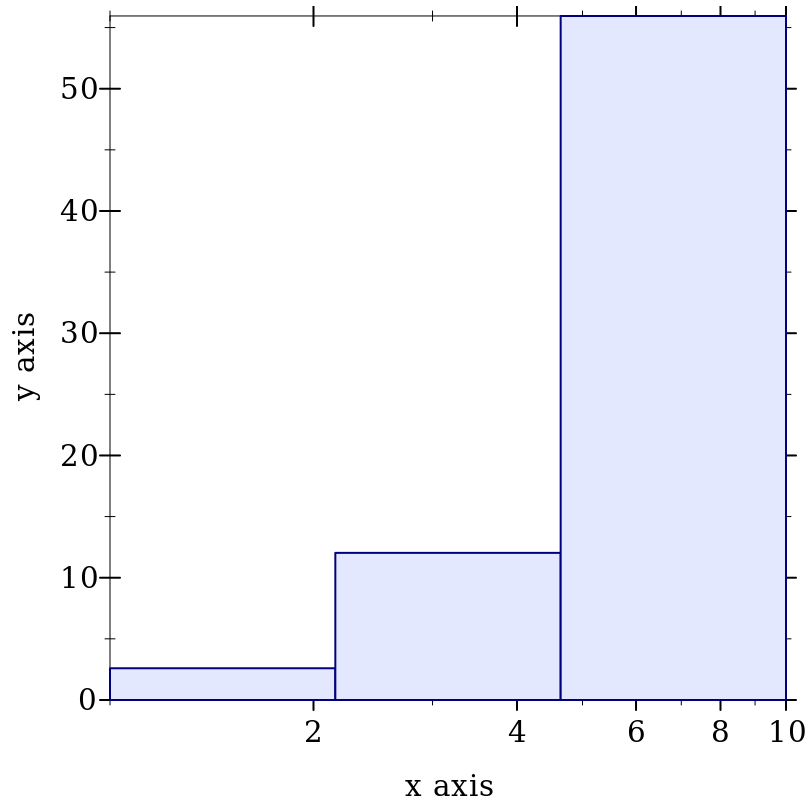procedure

(kde xs h [ws])
 (-> real? real?) (or/c rational? #f) (or/c rational? #f)
xs : (listof real?)
h : (>/c 0)
ws : (or/c (listof (>=/c 0)) #f) = #f
Given optionally weighted samples and a kernel bandwidth, returns a function representing a kernel density estimate, and bounds, outside of which the density estimate is zero. Used by density.

#### 7.3Plot Colors and Styles

procedure

 (color-seq c1 c2 num [ #:start? start? #:end? end?])
(listof (list/c real? real? real?))
c1 : color/c
c2 : color/c
num : exact-nonnegative-integer?
start? : boolean? = #t
end? : boolean? = #t
Interpolates between colors—red, green and blue components separately—using linear-seq. The #:start? and #:end? keyword arguments work as in linear-seq.

Example:

 > (plot (contour-intervals (λ (x y) (+ x y)) -2 2 -2 2 #:levels 4 #:contour-styles '(transparent) #:colors (color-seq "red" "blue" 5)))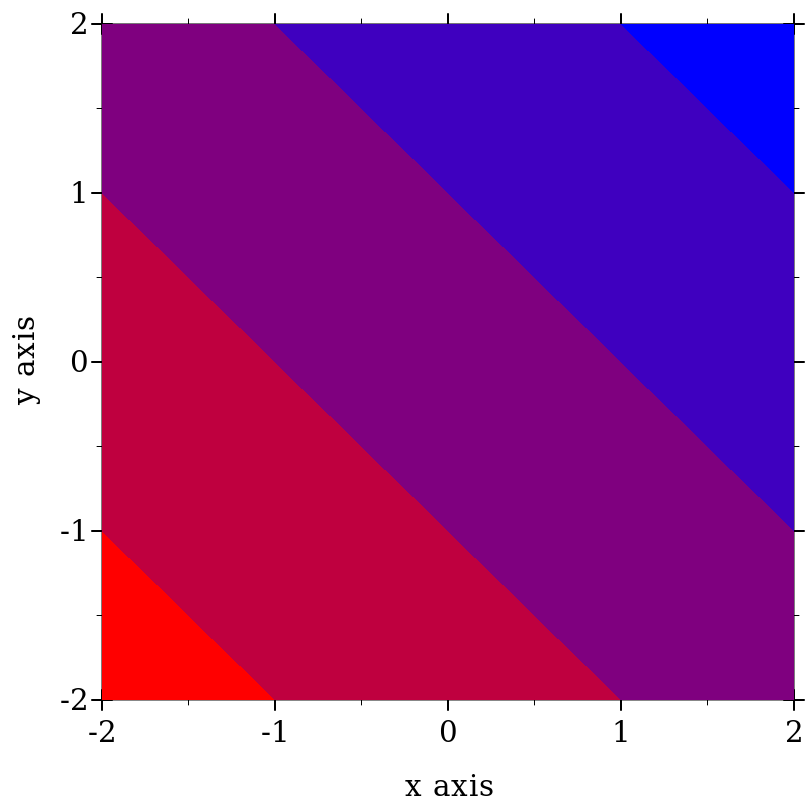procedure

 (color-seq* colors num [ #:start? start? #:end? end?])
(listof (list/c real? real? real?))
colors : (listof color/c)
num : exact-nonnegative-integer?
start? : boolean? = #t
end? : boolean? = #t
Interpolates between colors—red, green and blue components separately—using linear-seq*. The #:start? and #:end? keyword arguments work as in linear-seq.

Example:

 > (plot (contour-intervals (λ (x y) (+ x y)) -2 2 -2 2 #:levels 4 #:contour-styles '(transparent) #:colors (color-seq* '(red white blue) 5)))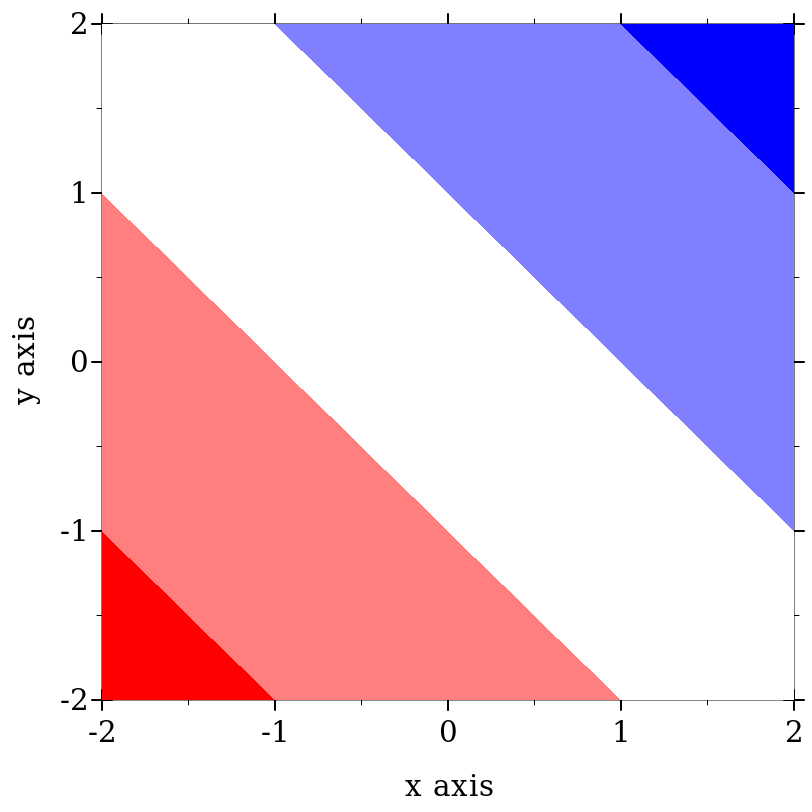procedure c : color/c
Converts a non-integer plot color to an RGB triplet.

Symbols are converted to strings, and strings are looked up in a color-database<%>. Lists are unchanged, and color% objects are converted straightforwardly.

Examples:

 > (->color 'navy) '(36 36 140) > (->color "navy") '(36 36 140) > (->color '(36 36 140)) '(36 36 140) > (->color (make-object color% 36 36 140)) '(36 36 140)

This function does not convert integers to RGB triplets, because there is no way for it to know whether the color will be used for a pen or for a brush. Use ->pen-color and ->brush-color to convert integers.

 procedure c : plot-color/c
Converts a line color to an RGB triplet. This function interprets integer colors as darker and more saturated than ->brush-color does.

Non-integer colors are converted using ->color. Integer colors are chosen for good pairwise contrast, especially between neighbors. Integer colors repeat starting with 128.

Examples:

> (equal? (->pen-color 0) (->pen-color 8))

#f

 > (plot (contour-intervals (λ (x y) (+ x y)) -2 2 -2 2 #:levels 7 #:contour-styles '(transparent) #:colors (map ->pen-color (build-list 8 values))))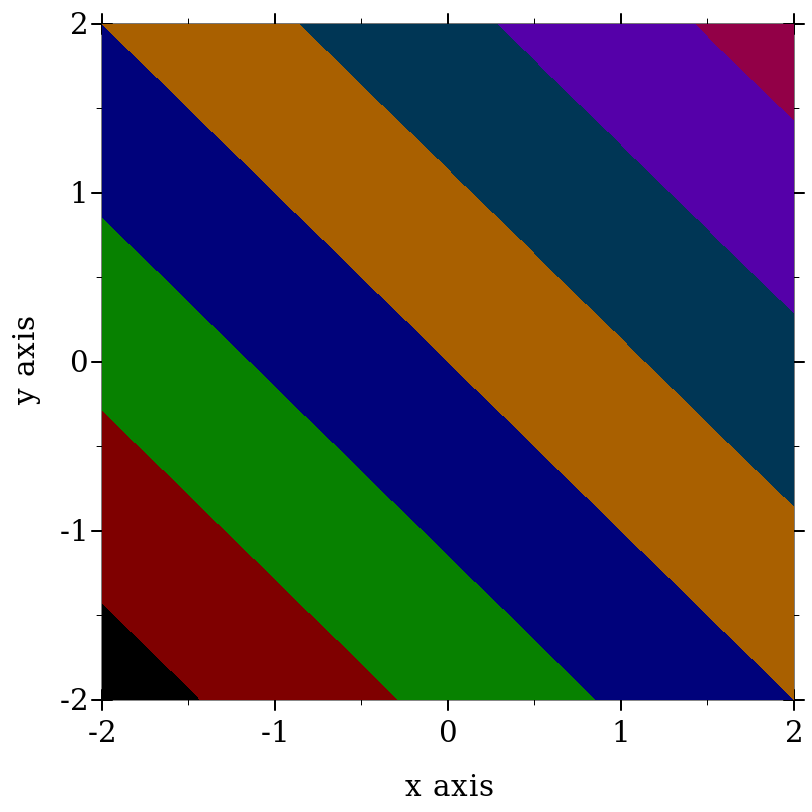procedure c : plot-color/c
Converts a fill color to an RGB triplet. This function interprets integer colors as lighter and less saturated than ->pen-color does.

Non-integer colors are converted using ->color. Integer colors are chosen for good pairwise contrast, especially between neighbors. Integer colors repeat starting with 128.

Examples:

> (equal? (->brush-color 0) (->brush-color 8))

#f

 > (plot (contour-intervals (λ (x y) (+ x y)) -2 2 -2 2 #:levels 7 #:contour-styles '(transparent) #:colors (map ->brush-color (build-list 8 values))))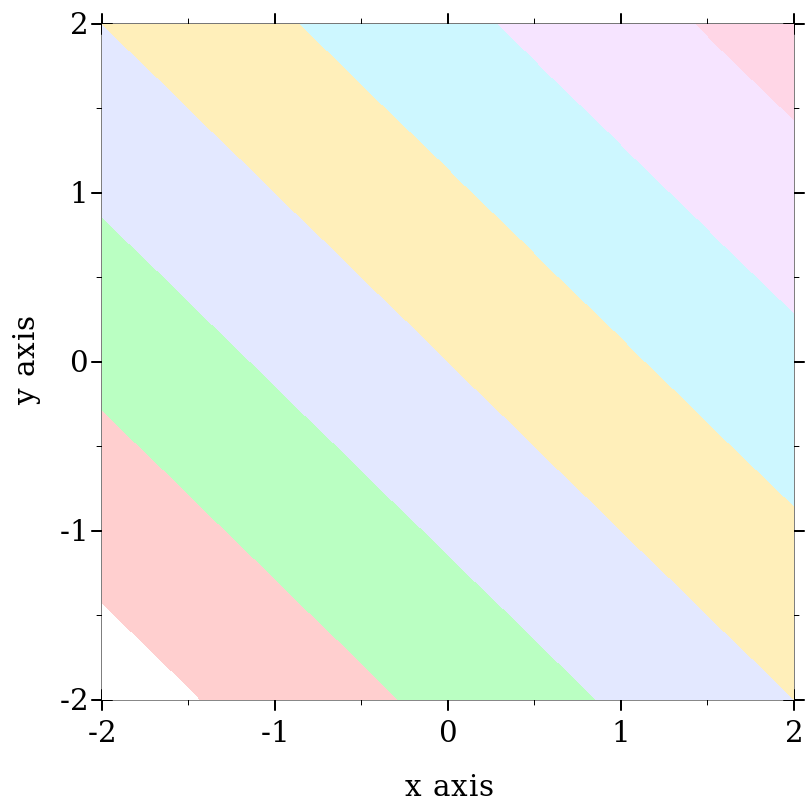In the above example, mapping ->brush-color over the list is actually unnecessary, because contour-intervals uses ->brush-color internally to convert fill colors.

The function-interval function generally plots areas using a fill color and lines using a line color. Both kinds of color have the default value 3. The following example reverses the default behavior; i.e it draws areas using line color 3 and lines using fill color 3:
 > (plot (function-interval sin (λ (x) 0) -4 4 #:color (->pen-color 3) #:line1-color (->brush-color 3) #:line2-color (->brush-color 3) #:line1-width 4 #:line2-width 4))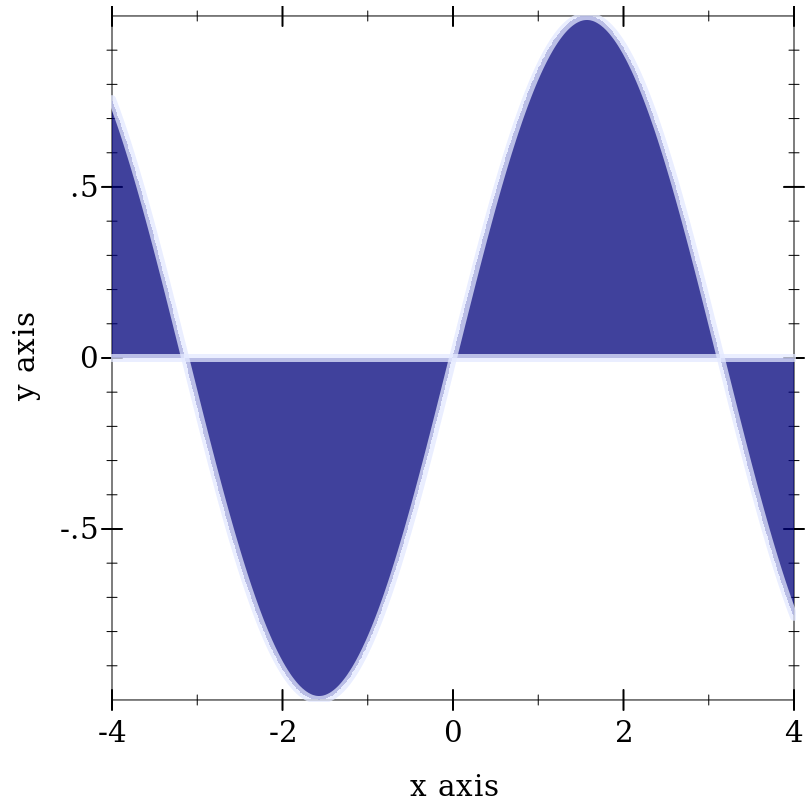procedure s : plot-pen-style/c
Converts a symbolic pen style or a number to a symbolic pen style. Symbols are unchanged. Integer pen styles repeat starting at 5.

Examples:

 > (eq? (->pen-style 0) (->pen-style 5)) #t > (map ->pen-style '(0 1 2 3 4)) '(solid dot long-dash short-dash dot-dash)

 procedure s : plot-brush-style/c
Converts a symbolic brush style or a number to a symbolic brush style. Symbols are unchanged. Integer brush styles repeat starting at 7.

Examples:

 > (eq? (->brush-style 0) (->brush-style 7)) #t > (map ->brush-style '(0 1 2 3)) '(solid bdiagonal-hatch fdiagonal-hatch crossdiag-hatch) > (map ->brush-style '(4 5 6)) '(horizontal-hatch vertical-hatch cross-hatch)

#### 7.4Plot-Specific Math

##### 7.4.1Real Functions

 procedure θ : real? r : real?
Converts 2D polar coordinates to 3D cartesian coordinates.

 procedure θ : real? ρ : real? r : real?
Converts 3D polar coordinates to 3D cartesian coordinates. See parametric3d for an example of use.

 procedure b : (and/c exact-integer? (>=/c 2)) x : (>/c 0)
Like (ceiling (/ (log x) (log b))), but ceiling-log/base is not susceptible to floating-point error.

Examples:

 > (ceiling (/ (log 100) (log 10))) 2.0 > (ceiling-log/base 10 100) 2 > (ceiling (/ (log 1/1000) (log 10))) -2.0 > (ceiling-log/base 10 1/1000) -3
Various number-formatting functions use this.

 procedure b : (and/c exact-integer? (>=/c 2)) x : (>/c 0)
Like (floor (/ (log x) (log b))), but floor-log/base is not susceptible to floating-point error.

Examples:

 > (floor (/ (log 100) (log 10))) 2.0 > (floor-log/base 10 100) 2 > (floor (/ (log 1000) (log 10))) 2.0 > (floor-log/base 10 1000) 3
This is a generalization of order-of-magnitude.

 procedure x : (or/c rational? #f)
Returns #f if x is #f; otherwise (inexact->exact x). Use this to convert interval endpoints, which may be #f, to exact numbers.

##### 7.4.2Vector Functions

 procedure(v+ v1 v2) → (vectorof real?) v1 : (vectorof real?) v2 : (vectorof real?)
 procedure(v- v1 v2) → (vectorof real?) v1 : (vectorof real?) v2 : (vectorof real?)
 procedure(vneg v) → (vectorof real?) v : (vectorof real?)
 procedure(v* v c) → (vectorof real?) v : (vectorof real?) c : real?
 procedure(v/ v c) → (vectorof real?) v : (vectorof real?) c : real?
Vector arithmetic. Equivalent to vector-mapp-ing arithmetic operators over vectors, but specialized so that 2- and 3-vector operations are much faster.

Examples:

 > (v+ #(1 2) #(3 4)) '#(4 6) > (v- #(1 2) #(3 4)) '#(-2 -2) > (vneg #(1 2)) '#(-1 -2) > (v* #(1 2 3) 2) '#(2 4 6) > (v/ #(1 2 3) 2) '#(1/2 1 3/2)

 procedure(v= v1 v2) → boolean? v1 : (vectorof real?) v2 : (vectorof real?)
Like equal? specialized to numeric vectors, but compares elements using =.

Examples:

 > (equal? #(1 2) #(1 2)) #t > (equal? #(1 2) #(1.0 2.0)) #f > (v= #(1 2) #(1.0 2.0)) #t

 procedure(vcross v1 v2) → (vector/c real? real? real?) v1 : (vector/c real? real? real?) v2 : (vector/c real? real? real?)
Returns the right-hand vector cross product of v1 and v2.

Examples:

 > (vcross #(1 0 0) #(0 1 0)) '#(0 0 1) > (vcross #(0 1 0) #(1 0 0)) '#(0 0 -1) > (vcross #(0 0 1) #(0 0 1)) '#(0 0 0)

 procedure(vcross2 v1 v2) → real? v1 : (vector/c real? real?) v2 : (vector/c real? real?)
Returns the signed area of the 2D parallelogram with sides v1 and v2. Equivalent to (vector-ref (vcross (vector-append v1 #(0)) (vector-append v2 #(0))) 2) but faster.

Examples:

 > (vcross2 #(1 0) #(0 1)) 1 > (vcross2 #(0 1) #(1 0)) -1

 procedure(vdot v1 v2) → real? v1 : (vectorof real?) v2 : (vectorof real?)
Returns the dot product of v1 and v2.

 procedure(vmag^2 v) → real? v : (vectorof real?)
Returns the squared magnitude of v. Equivalent to (vdot v v).

 procedure(vmag v) → real? v : (vectorof real?)
Returns the magnitude of v. Equivalent to (sqrt (vmag^2 v)).

 procedure(vnormalize v) → (vectorof real?) v : (vectorof real?)
Returns a normal vector in the same direction as v. If v is a zero vector, returns v.

Examples:

 > (vnormalize #(1 1 0)) '#(0.7071067811865475 0.7071067811865475 0) > (vnormalize #(1 1 1)) '#(0.5773502691896258 0.5773502691896258 0.5773502691896258) > (vnormalize #(0 0 0.0)) '#(0 0 0.0)

 procedure(vcenter vs) → (vectorof real?) vs : (listof (vectorof real?))
Returns the center of the smallest bounding box that contains vs.

Example:

 > (vcenter '(#(1 1) #(2 2))) '#(3/2 3/2)

 procedure v : (vectorof real?)
Returns #t if every element of v is rational?.

Examples:

 > (vrational? #(1 2)) #t > (vrational? #(+inf.0 2)) #f > (vrational? #(#f 1)) vrational?: contract violation expected: Real given: #f in: an element of the 1st argument of (-> (vectorof Real) any) contract from: /plot-lib/plot/private/common/math.rkt blaming: top-level (assuming the contract is correct) at: /plot-lib/plot/private/common/math.rkt:330.9

##### 7.4.3Intervals and Interval Functions

struct

 (struct ivl (min max)
#:extra-constructor-name make-ivl)
min : real?
max : real?
Represents a closed interval.

An interval with two real-valued endpoints always contains the endpoints in order:
 > (ivl 0 1) (ivl 0 1) > (ivl 1 0) (ivl 0 1)

An interval can have infinite endpoints:
 > (ivl -inf.0 0) (ivl -inf.0 0) > (ivl 0 +inf.0) (ivl 0 +inf.0) > (ivl -inf.0 +inf.0) (ivl -inf.0 +inf.0)

Functions that return rectangle renderers, such as rectangles and discrete-histogram3d, accept vectors of ivls as arguments. The ivl struct type is also provided by plot so users of such renderers do not have to require plot/utils.

 procedure i : any/c
Returns #t if i is an interval and each of its endpoints is rational?.

Example:

 > (map rational-ivl? (list (ivl -1 1) (ivl -inf.0 2) 'bob)) '(#t #f #f)

 procedure(bounds->intervals xs) → (listof ivl?) xs : (listof real?)
Given a list of points, returns intervals between each pair.

Use this to construct inputs for rectangles and rectangles3d.

Example:

 > (bounds->intervals (linear-seq 0 1 5)) (list (ivl 0 1/4) (ivl 1/4 1/2) (ivl 1/2 3/4) (ivl 3/4 1))

 procedure(clamp-real x i) → real? x : real? i : ivl?

#### 7.5Dates and Times

 procedure x : (or/c plot-time? date? date*? sql-date? sql-time? sql-timestamp?)
Converts various date/time representations into UTC seconds, respecting time zone offsets.

For dates, the value returned is the number of seconds since a system-dependent UTC epoch. See date-ticks for more information.

To plot a time series using dates pulled from an SQL database, simply set the relevant axis ticks (probably plot-x-ticks) to date-ticks, and convert the dates to seconds using datetime->real before passing them to lines. To keep time zone offsets from influencing the plot, set them to 0 first.

struct

 (struct plot-time (second minute hour day)
#:extra-constructor-name make-plot-time)
second : (and/c (>=/c 0) (</c 60))
minute : (integer-in 0 59)
hour : (integer-in 0 23)
day : exact-integer?
A time representation that accounts for days, negative times (using negative days), and fractional seconds.

Plot (specifically time-ticks) uses plot-time internally to format times, but because renderer-producing functions require only real values, user code should not need it. It is provided just in case.

 procedure t : plot-time?
 procedure s : real?
Convert plot-times to real seconds, and vice-versa.

Examples:

 > (define (plot-time+ t1 t2) (seconds->plot-time (+ (plot-time->seconds t1) (plot-time->seconds t2))))
 > (plot-time+ (plot-time 32 0 12 1) (plot-time 32 0 14 1))

(plot-time 4 1 2 3)